# Interior Angles of Polygons

### An Interior Angle is an angle inside a shapeAnother example:## Triangles

The Interior Angles of a Triangle add up to 180°

Let's try a triangle: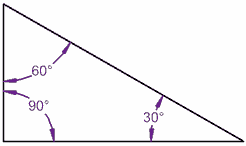90° + 60° + 30° = 180°

It works for this triangle

Now tilt a line by 10°: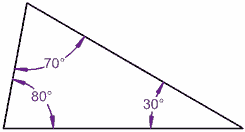80° + 70° + 30° = 180°

It still works!
One angle went up by 10°,
and the other went down by 10°

(A Quadrilateral has 4 straight sides)

Let's try a square: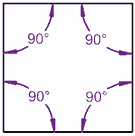90° + 90° + 90° + 90° = 360°

A Square adds up to 360°

Now tilt a line by 10°: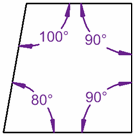80° + 100° + 90° + 90° = 360°

It still adds up to 360°

### Because there are 2 triangles in a square ...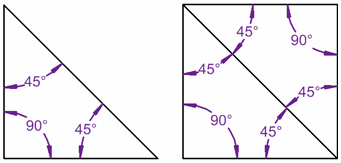The interior angles in a triangle add up to 180° ...

... and for the square they add up to 360° ...

... because the square can be made from two triangles!

## Pentagon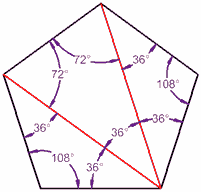A pentagon has 5 sides, and can be made from three triangles, so you know what ...

... its interior angles add up to 3 × 180° = 540°

And when it is regular (all angles the same), then each angle is 540° / 5 = 108°

(Exercise: make sure each triangle here adds up to 180°, and check that the pentagon's interior angles add up to 540°)

The Interior Angles of a Pentagon add up to 540°

## The General Rule

Shape Sides Sum of Shape Each Angle Interior Angles If it is a Regular Polygon (all sides are equal, all angles are equal) Triangle 3 180°60° Quadrilateral 4 360°90° Pentagon 5 540°108° Hexagon 6 720°120° Heptagon (or Septagon) 7 900°128.57...° Octagon 8 1080°135° Nonagon 9 1260°140° ... ... .. ... ... Any Polygon n (n−2) × 180°(n−2) × 180° / n

So the general rule is:

Sum of Interior Angles = (n−2) × 180°

Each Angle (of a Regular Polygon) = (n−2) × 180° / n

Perhaps an example will help:

### Example: What about a Regular Decagon (10 sides) ?Sum of Interior Angles = (n−2) × 180°
= (10−2) × 180°
= 8 × 180°
= 1440°

And for a Regular Decagon:

Each interior angle = 1440°/10 = 144°

Note: Interior Angles are sometimes called "Internal Angles"